# ML Aggarwal Class 6 Solutions for ICSE Maths Chapter 7 Decimals Ex 7.1

## ML Aggarwal Class 6 Solutions for ICSE Maths Chapter 7 Decimals Ex 7.1

Question 1.
Write each of the following decimal numbers in words:
(i) 30.5
(ii) 0.03
(iii) 108.56
(iv) 47.20
(v) 5.008
(vi) 26.039
Solution:Question 2.
Write each of the following decimal numbers in the place value table :
(i) 4.2
(ii) 0.3
(iii) 205.9
(iv) 0.29
(v) 2.08
(vi) 7200.812
(vii) 38.007
Solution:Question 3.
Write the following decimal numbers in the expanded form :
(i) 123.7
(ii) 43.06
(iii) 509.306
Solution: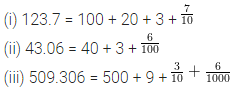Question 4.
Write each of the following as a decimal number :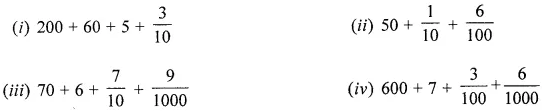Solution: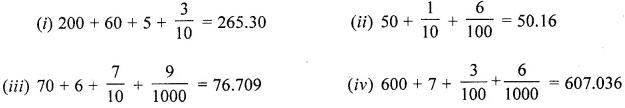Question 5.
Write each of the following as decimals:
(i) Two ones and five tenths
(ii) Two tens and nine tenths
(iii) Six hundred point eight
(iv) Two hundred five anf five hundredths
(v) Seven and fifteen thousandths
Solution: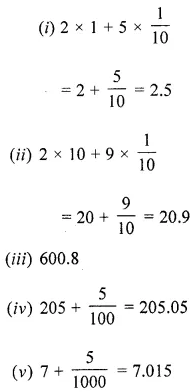Question 6.
Write the number given in the following place value table in decimal form: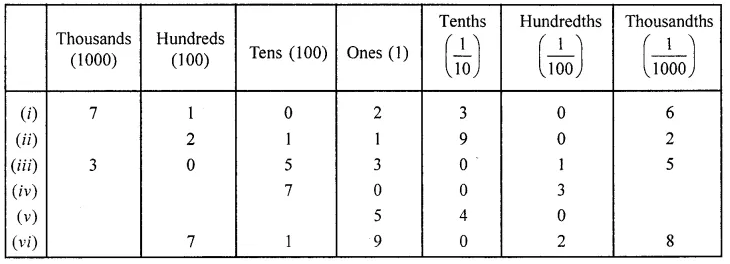Solution:Question 7.
Show the following decimal numbers on the number line:
(i) 0.4
(ii) 1.9
(iii) 1.1
(iv) 2.5
Solution: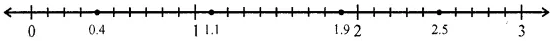Question 8.
Write the decimal numbers represented by the points A, B, C and D on the given number line:Solution:Question 9.
Between which two numbers in tenths place on the number line does each of the given number lie?
(i) 0.06
(ii) 0.45
(iii) 0.66
(iv) 0.92
Solution: